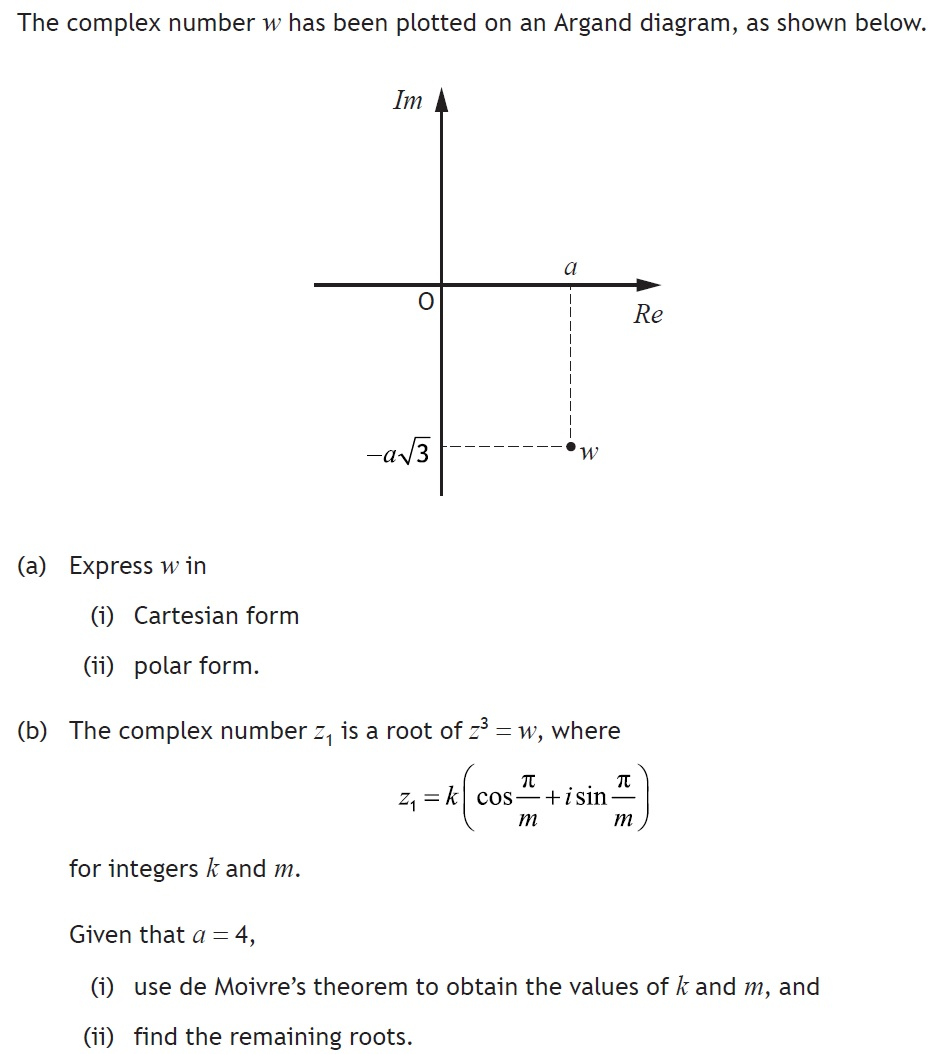# Practice Questions – Complex Numbers

Q1. The complex numbers  ${z}_{1}$ and  ${z}_{2}$ are given by  ${z}_{1}=\sqrt{2}+\sqrt{2}i$ and  ${z}_{2}=\sqrt{3}–i$

(i) Find the modulus and argument of each of  ${z}_{1}$ and  ${z}_{2}$ .  [6 marks]
(ii) Plot the points representing each of ${z}_{1}$${z}_{2}$ and  ${z}_{1}+{z}_{2}$ on an Argand diagram. [3 marks]
(iii) Hence find the exact value of  $\mathrm{tan}\frac{\mathrm{\pi }}{24}$ . [5 marks]

Q2.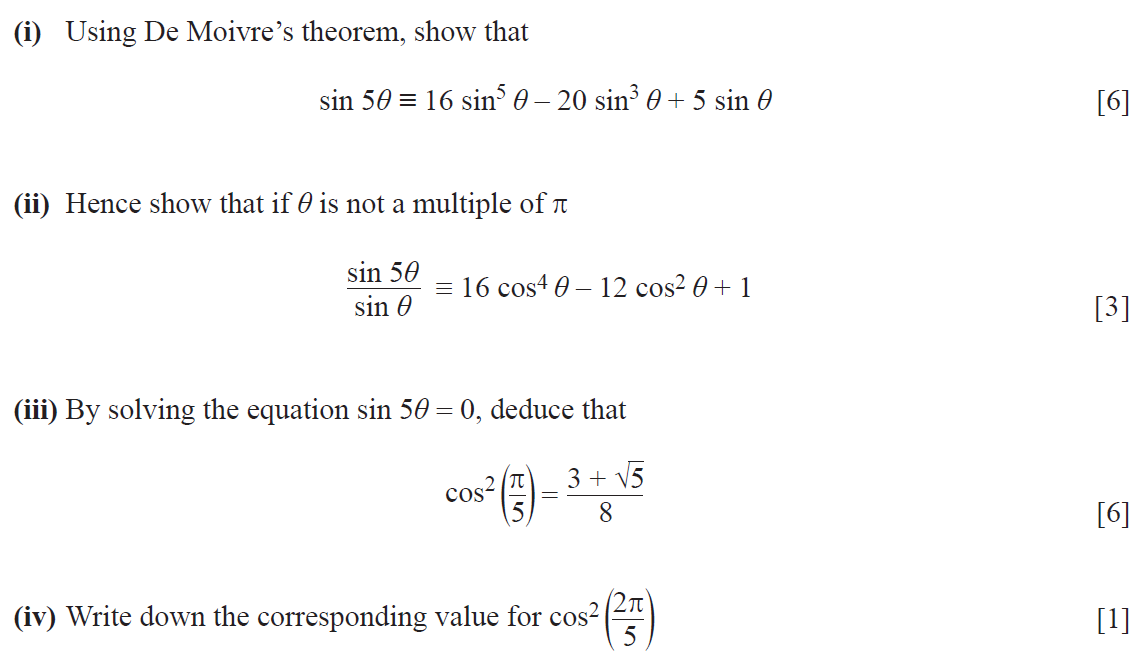Q3.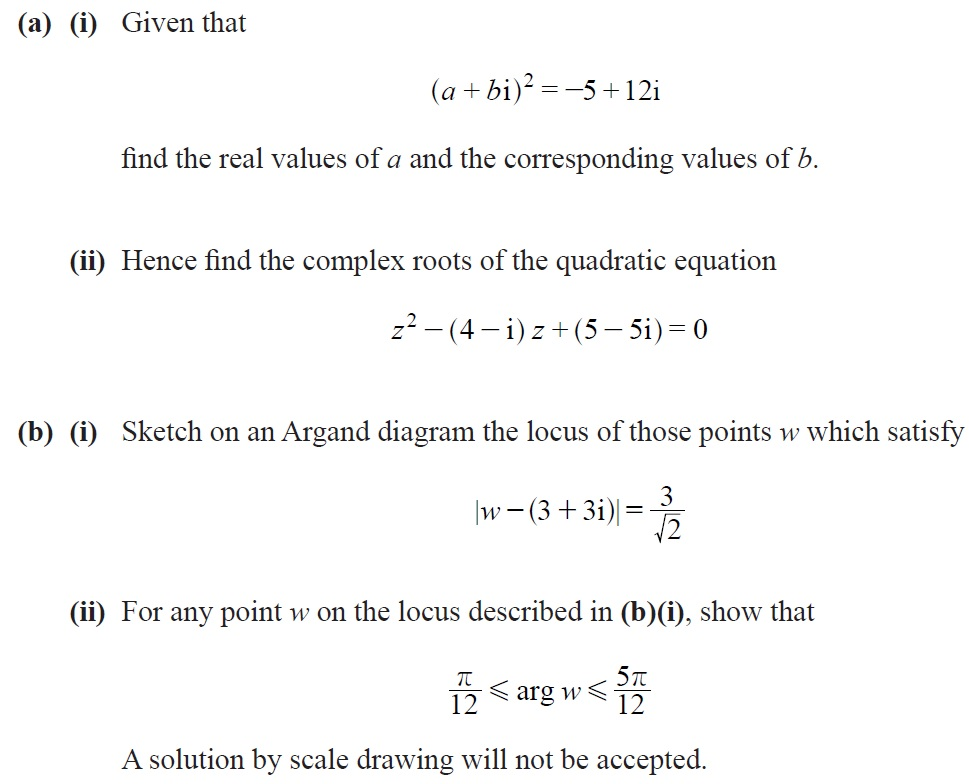Q4.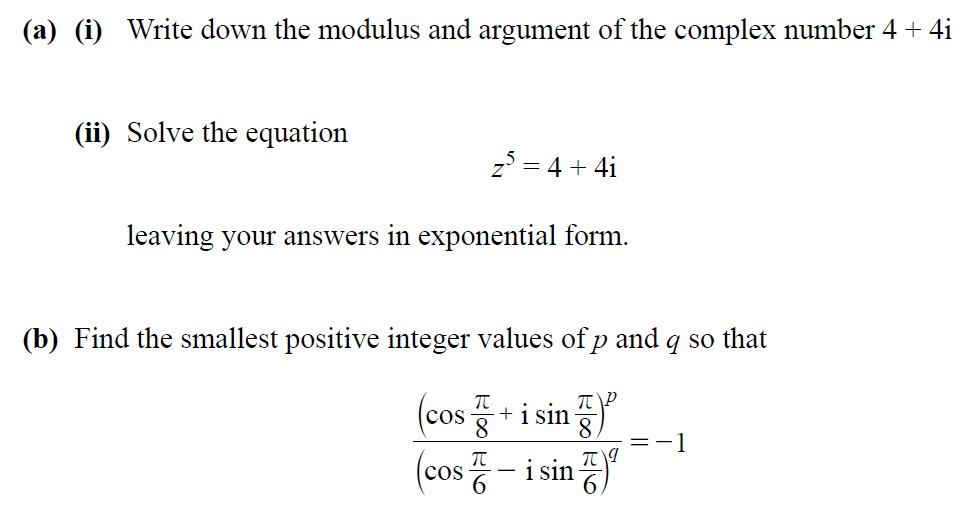Q5.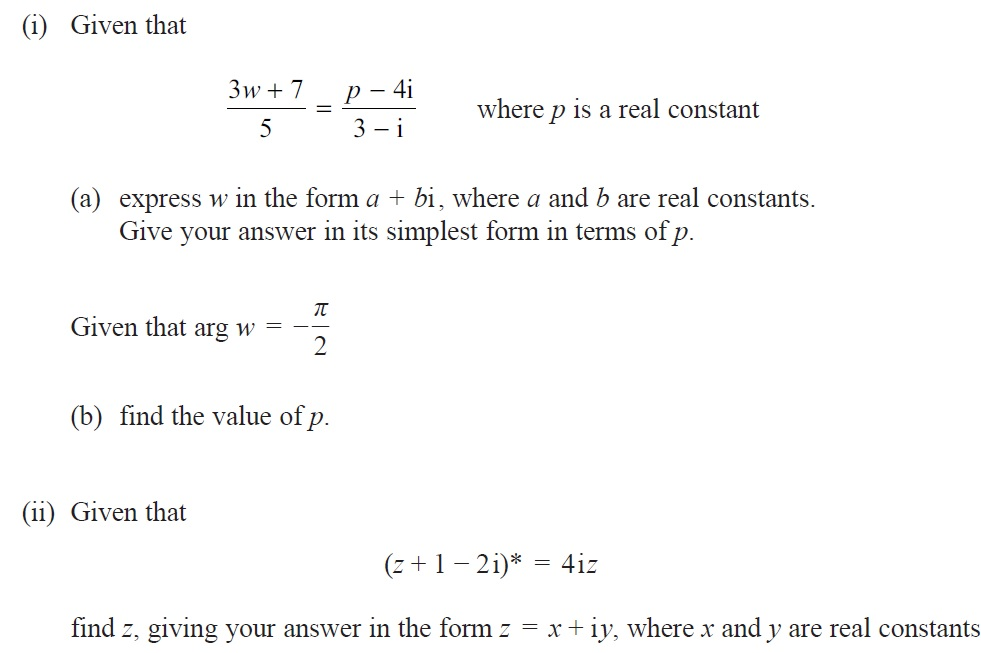Q6. (i) The complex number  $z$ is such that ${z}^{2}=1+i\sqrt{3}$ . Find the two possible values of  in the forma $a+ib$ , where  $a$ and $b$ are exact real numbers. [5 marks]
(ii) With the value of  $z$ from part (i)such that the real part of $z$ is positive, show on an Argand diagram the points A and B representing  $z$ and ${z}^{2}$ respectively. [2 marks]
(iii) Specify two transformations which together map the line segment OA to the line segment OB, where O is the origin. [4 marks]

Q7.